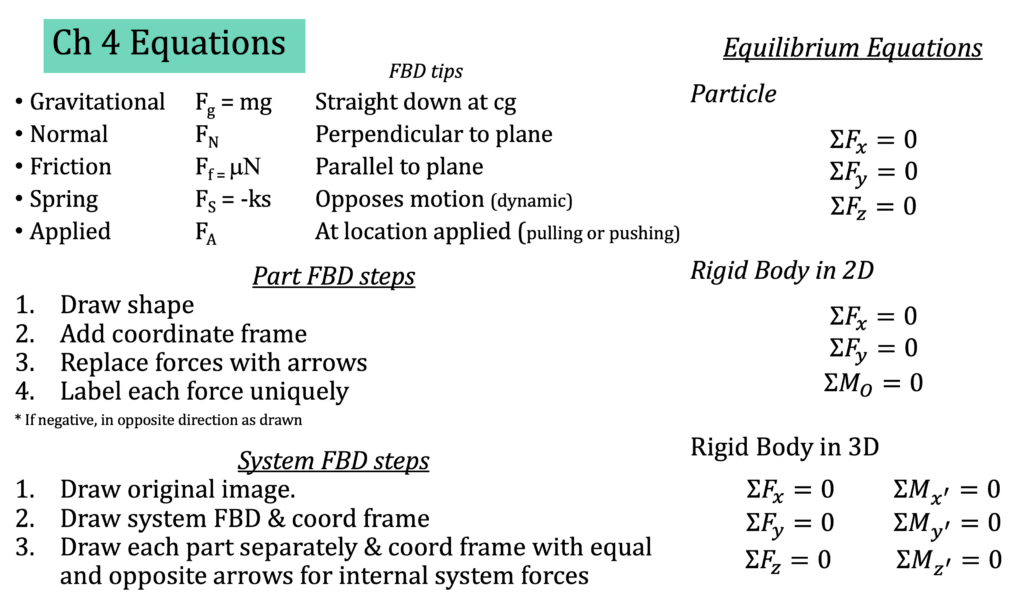# Chapter 4: Rigid Bodies

This is arguably the most fundamental chapter for Statics. Learn these concepts and the next two chapters will make a lot of sense. Without this chapter, the next chapters will be much more confusing. When people talk about Statics, this chapter contains the concepts they are talking about. You will use free-body diagrams and the equilibrium equations in many other courses. Here are the sections in this Chapter:

Here are the important equations for this chapter.